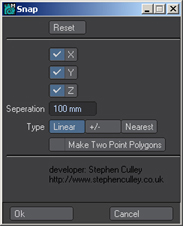## Feedback

Please leave feedback and comments. I am always interested to hear how people get on using these LScripts!

## Friday, 10 June 2011

### LScript - Modeler_SnapLScript (Modeler) to snaps points in different ways to other points. It can align points in linear, either side of currently selected points or by making points snap to there nearest neighbour a set distance away.

Compatible with Newtek LightWave 9.6 and above.

```// LScript Modeler - www.StephenCulley.co.uk
//

/*
LScript Modeler - Snap

Modeler_Snap.ls

*/

@version 2.2
@warnings
@script modeler
@name *Snap

// Title
sTitle = "*Snap";

// Version
sVersion = "v1.0";

ctrl_c0,ctrl_c1,ctrl_c2,ctrl_c3,ctrl_c4,ctrl_c5;

main
{

// Store
bAxisX = recall("bAxisX",true);
bAxisY = recall("bAxisY",true);
bAxisZ = recall("bAxisZ",true);
nSeperation = recall("nSeperation",0.1);
iType = recall("iType",1);
bTwoPointPolygon = recall("bTwoPointPolygon",false);

reqbegin(sTitle + " " + sVersion);

// Reset
ctrl_res0 = ctlbutton("Reset",50,"button_reset"); // Button Reset
ctlsep();

// Control
ctrl_c0 = ctlcheckbox("X", bAxisX);
ctrl_c1 = ctlcheckbox("Y", bAxisY);
ctrl_c2 = ctlcheckbox("Z", bAxisZ);
ctrl_c3 = ctldistance("Seperation",nSeperation);
ctrl_c4 = ctlchoice("Type",iType,@"Linear","+/-","Nearest"@);
ctrl_c5 = ctlcheckbox("Make Two Point Polygons", bTwoPointPolygon);

// Developer
ctlsep();
ctrl_dev0 = ctltext("","developer: Stephen Culley","http://www.stephenculley.co.uk");

return if !reqpost();

bAxisX = getvalue(ctrl_c0); // X
bAxisY = getvalue(ctrl_c1); // Y
bAxisZ = getvalue(ctrl_c2); // Z
nSeperation = getvalue(ctrl_c3);
iType = getvalue(ctrl_c4);
bTwoPointPolygon = getvalue(ctrl_c5);

// Store
store("bAxisX",bAxisX);
store("bAxisY",bAxisY);
store("bAxisZ",bAxisZ);
store("nSeperation",nSeperation);
store("iType",iType);
store("bTwoPointPolygon",bTwoPointPolygon);

// Selection - Point (GLOBAL)
selmode(GLOBAL);
iPointCountBefore = pointcount();

// Selection - Point (DIRECT)
selmode(DIRECT);
iPointCount = pointcount();
if(iPointCount <= 1) error("None or not enough points selected.");

undogroupbegin(); // Undo

moninit(iPointCount,"Processing...");  // Progress Monitor

if(iType == 1) // Linear
{
editbegin();

vSnapPoint = pointinfo(points);
vTempPoint = vSnapPoint;
for(iCurrentPoint = 2; iCurrentPoint <= iPointCount; iCurrentPoint++)
{
if(bAxisX) vSnapPoint.x += nSeperation; // X
if(bAxisY) vSnapPoint.y += nSeperation; // Y
if(bAxisZ) vSnapPoint.z += nSeperation; // Z
pointmove(points[iCurrentPoint],vSnapPoint); // Move point

// Two Point Polygon
if(bTwoPointPolygon)
{
}

vTempPoint = vSnapPoint;

monstep(); // Progress Monitor
}

editend();
}

if(iType == 2) // +/-
{
editbegin();

vSnapPoint = pointinfo(points);
for(iCurrentPoint = 2; iCurrentPoint <= iPointCount; iCurrentPoint++)
{
vPoint = pointinfo(points[iCurrentPoint]);
vTempPoint = vSnapPoint;
if(bAxisX) vTempPoint.x = snap(vPoint.x,vSnapPoint.x,nSeperation); // X
if(bAxisY) vTempPoint.y = snap(vPoint.y,vSnapPoint.y,nSeperation); // Y
if(bAxisZ) vTempPoint.z = snap(vPoint.z,vSnapPoint.z,nSeperation); // Z
pointmove(points[iCurrentPoint],vTempPoint); // Move point

// Two Point Polygon
if(bTwoPointPolygon)
{
}

vSnapPoint = vTempPoint;

monstep(); // Progress Monitor
}

editend();
}

if(iType == 3) // Nearest
{
editbegin();

aPoints = pointinfo(points); // Add point to array
for(iCurrentPoint = 2; iCurrentPoint <= iPointCount; iCurrentPoint++)
{
vPoint = pointinfo(points[iCurrentPoint]);
vSnapPoint = aPoints;
Distance = distance3D(vPoint,vSnapPoint);
for(iCurrentPoint = 1; iCurrentPoint <= aPoints.size(); iCurrentPoint++)
{
DistanceNew = distance3D(vPoint,aPoints[iCurrentPoint]);
if(DistanceNew < Distance)
{
vSnapPoint = aPoints[iCurrentPoint];
Distance = DistanceNew;
}
}
vTempPoint = vSnapPoint;
if(bAxisX) vTempPoint.x = snap(vPoint.x,vSnapPoint.x,nSeperation); // X
if(bAxisY) vTempPoint.y = snap(vPoint.y,vSnapPoint.y,nSeperation); // Y
if(bAxisZ) vTempPoint.z = snap(vPoint.z,vSnapPoint.z,nSeperation); // Z
pointmove(points[iCurrentPoint],vTempPoint); // Move point

// Two Point Polygon
if(bTwoPointPolygon)
{
}

aPoints[iCurrentPoint] = vTempPoint; // Add to array

monstep(); // Progress Monitor
}

editend();

monend(); // Progress Monitor
}

// Selection - Point (GLOBAL)
selmode(GLOBAL);
iPointCountAfter = pointcount();
if((iPointCountAfter - iPointCountBefore) > 0)
{
for(s = 1; s <= (iPointCountAfter - iPointCountBefore) ; s++){aSelection[s] = s + iPointCountBefore;}
selmode(USER);
selpoint(SET, POINTNDX, aSelection);

mergepoints(0.0); // Merge Points
}

undogroupend(); // Undo

}

button_reset
{
setvalue(ctrl_c0,true); // X
setvalue(ctrl_c1,true); // Y
setvalue(ctrl_c2,true); // Z
setvalue(ctrl_c3,0.1); // Seperation
setvalue(ctrl_c4,1); // Type
setvalue(ctrl_c5,false); // Two Point Polygon
}

// SNAP

snap: n1,n2,n
{
if(n1 < n2){return(n2 - n);}
else
{return(n2 + n);}
}

// VECTOR

distance3D: v1, v2 // n
{
// Vector
return(sqrt(((v2.x - v1.x) * (v2.x - v1.x)) +
((v2.y - v1.y) * (v2.y - v1.y)) +
((v2.z - v1.z) * (v2.z - v1.z))));
}```
All scripts available at my Google Drive at

1.Thanks so much!

2.Hi. I'm trying out this script, and I was not sure how it works. I have 3 points that I want to line up into a "straight" alignment in 3d space. Is this possible? Do I deselect the X, Y, and Z axis?

3.just deselect the axis you don't want. make sure the first selected point is the point you want to align with, all other points selected after will then align with the first selected. Hope this helps.

4.Steve do you know of a script which will allow me to align points based on the first and last selected points irrespective of XYZ.

1.Not that I have seen.. Would it be like align to nearest position on line or distance % from either end? Im curious how you decide where to position or is it simply to get it on the same vectors?

5.I would like all the points to align in a row between the first and last points. I find i have been doing allot of diagonal modeling lately and whilst align to last points position on x,y,z, its no good if you have a diagonal plane or such. I mean the plane is a normal oblong its just orientated in 3d space diagonally. Yes i could rotate it and then back but i have some buildings that are pretty poly heavy and rotating them all the time is a pain. Of course if we had some really good snap tools in modeler this would help.